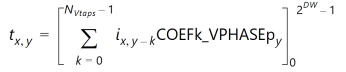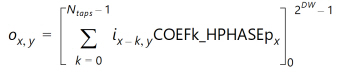# Convert 4:2:0 to 4:4:4 - 2.4 English

## Video Processing Subsystem Product Guide (PG231)

Document ID
PG231
Release Date
2022-10-19
Version
2.4 English

This conversion performs interpolation both vertically and horizontally. This is equivalent to a 2D separable filter implemented by cascading the 4:2:0 to 4:2:2 block and the 4:2:2 to 4:4:4 block. Quantized vertical filter results are filtered by the horizontal filter, which in turn quantizes results back to the [0 .. 2DW-1] range. (DW is the Data Width or number of bits per video component.)

Intermediate 4:2:2 chroma values are computed using This Equation . The resulting computation is shown in This Equation .

Equation 3-14Next, the values are filtered according to This Equation . The resulting computation is shown in This Equation .

Equation 3-15The predefined filter coefficients are the same as defined in Convert 4:2:0 to 4:2:2 and Convert 4:2:2 to 4:4:4. In the vertical direction, one input sample is replicated, and the other is interpolated with the filter [0.5 0.5]. The same then happens in the horizontal direction.